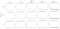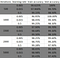# Using Logistic Regression for Image Classification

Machine Learning Project

# SYSTEM REQUIREMENTS

• Basic python programming skills
• Basic knowledge of logistic regression
• Numpy python library
• Pandas python library
• Matplotlib python library
`import pandas as pdimport numpy as npimport matplotlib.pyplot as plt`

# PROBLEM STATEMENT

The idea of this project is to develop and train a model that is able to take the pixel values of a digit and identify if it is an image of the digit one or not.

# SET UP OF DATA

`df_x= pd.read_excel('dataset.xlsx', 'X', header=None)df_x.head()df_y= pd.read_excel('dataset.xlsx', 'Y', header=None)df_y.head()`
`y = df_yfor i in range(len(y)):   if y[i] != 1:      y[i] = 0y = pd.DataFrame(y)`
`x_train = df_x.iloc[0:4000].Tx_test = df_x.iloc[4000:].Tx_train = np.array(x_train)x_test = np.array(x_test)y_train = y.iloc[0:4000].Ty_test = y.iloc[4000:].Ty_train = np.array(y_train)y_test = np.array(y_test)`

# FORMULAS

We start from the basic linear regression formula:

• x is the input variable
• w and b will be initialized as zeros and they will be modified while training the model.
`def sigmoid(z):   s = 1/(1 + np.exp(-z))   return s`
`def initialize_with_zeros(dim):   w = np.zeros(shape=(dim, 1))   b = 0   return w, b`

# CODING THE MODEL

Once all the formulas are set, let’s put it all together in a function called propagate by adding the next lines in our code

`def propagate(w, b, X, Y):   #Find the number of training data   m = X.shape   #Calculate the predicted output   A = sigmoid(np.dot(w.T, X) + b)   #Calculate the cost function   cost = -1/m * np.sum(Y*np.log(A) + (1-Y) * np.log(1-A))   #Calculate the gradients   dw = 1/m * np.dot(X, (A-Y).T)   db = 1/m * np.sum(A-Y)      grads = {"dw": dw, "db": db}   return grads, cost`
• cost: Cost function
`def optimize(w, b, X, Y, num_iterations, learning_rate):   costs = []   #propagate function will run for a number of iterations   for i in range(num_iterations):      grads, cost = propagate(w, b, X, Y)      dw = grads["dw"]      db = grads["db"]            #Updating w and b by deducting the dw and db       #times learning rate from the previous w and b      w = w - learning_rate * dw      b = b - learning_rate * db      #Record the cost function value for each 100 iterations      if i % 100 == 0:         costs.append(cost)   #The final updated parameters   params = {"w": w,"b": b}   #The final updated gradients   grads = {"dw": dw,"db": db}      return params, grads, costs`
`def predict(w, b, X):   m = X.shape   w = w.reshape(X.shape, 1)   #Initializing an aray of zeros which has a size of the input   #These zeros will be replaced by the predicted output   Y_prediction = np.zeros((1, m))      #Calculating the predicted output using the sigmoid function   #This will return the values from 0 to 1   A = sigmoid(np.dot(w.T, X) + b)   #Iterating through A and predict an 1 if the value of A   #is greater than 0.5 and zero otherwise   for i in range(A.shape):      Y_prediction[:, i] = (A[:, i] > 0.5) * 1   return Y_prediction`
`def model(X_train, Y_train, X_test, Y_test, num_iterations, learning_rate):   #Initializing the w and b as zeros   w, b = initialize_with_zeros(X_train.shape)   #Best fit the training data   parameters, grads, costs = optimize(w, b, X_train, Y_train, num_iterations, learning_rate)      w = parameters["w"]   b = parameters["b"]   # Predicting the output for both test and training set   Y_prediction_test = predict(w, b, X_test)   Y_prediction_train = predict(w, b, X_train)   #Calculating the training and test set accuracy by comparing   #the predicted output and the original output   print("train accuracy: {} %".format(100 - np.mean(np.abs(Y_prediction_train - Y_train)) * 100))   print("test accuracy: {} %".format(100 - np.mean(np.abs(Y_prediction_test - Y_test)) * 100))   d = {"costs": costs,   "Y_prediction_test": Y_prediction_test,   "Y_prediction_train" : Y_prediction_train,   "w" : w,   "b" : b,   "learning_rate" : learning_rate,   "num_iterations": num_iterations}   return d`
`ni = 500 # num iterationslr = 0.005 # learning rated = model(x_train, y_train, x_test, y_test, ni, lr)`
`train accuracy: XX%test accuracy: XX%`
• Final parameters
• Predicted Outputs
• Learning Rate
• Number of iterarions used
`#Plot how cost function changed each updated w's and b'splt.figure(figsize=(7,5))plt.scatter(x = range(len(d['costs'])), y = d['costs'], color='black')plt.title('Scatter Plot of Cost Functions', fontsize=18)plt.ylabel('Costs', fontsize=12)plt.show()`Scatter Plot of Cost Functions with diferent learning rates and iterationsMultiple values of test and train accuracy depending on learning rate and iterations

# CONCLUSIONS

• With each iteration, the cost function went down as it should, that means the parameters w and b kept refining towards perfection.
• It is worth noting that increasing the value of the learning rate will get us a better % at training accuracy but it will be lower on test accuracy. For this particular case I recommend using 0.015 that had both percentages more even.
• To change the model for recognizing another digit apart from 1 you can go to the line where it changes all numbers that are not 1 to zero, but instead of 1 use the digit of your preference.
• Reducing the number of rows for training the model will underfit it and if we increase the number it will overfit it. But you can try and check how it behaves.